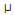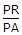# Electronics - Capacitors

### Exercise :: Capacitors - General Questions

71.

What will a 1F capacitor store?

 A. one coulomb of charge B. 6.24 x 1012 electrons C. one volt D. oneA of current

Explanation:

No answer description available for this question. Let us discuss.

72.

In an RC circuit, true power (PR) equals:

 A.B. VS × I C. I2R D. VT × RT

Explanation:

No answer description available for this question. Let us discuss.

73.

What is the power factor if the apparent power of a circuit is 5 W, while the true power is 4 W?

 A. 0.0 W B. 0.8 W C. 1.0 W D. 1.25 W

Explanation:

No answer description available for this question. Let us discuss.

74.

If the reactive power of a circuit is 50 mW while the apparent power is 64 mW, then what is the true power of the circuit?

 A. 14 mW B. 36 mW C. 40 mW D. 114 mW